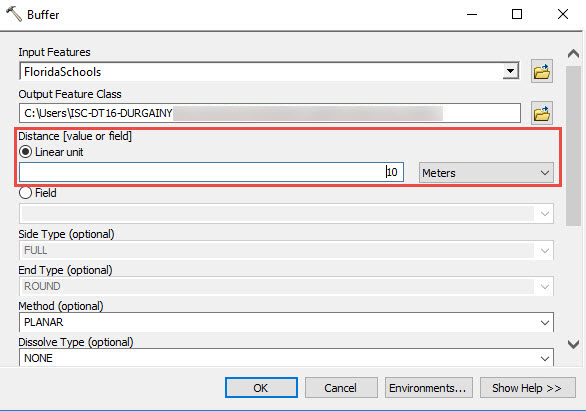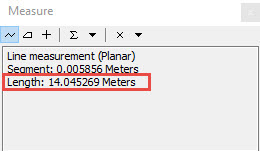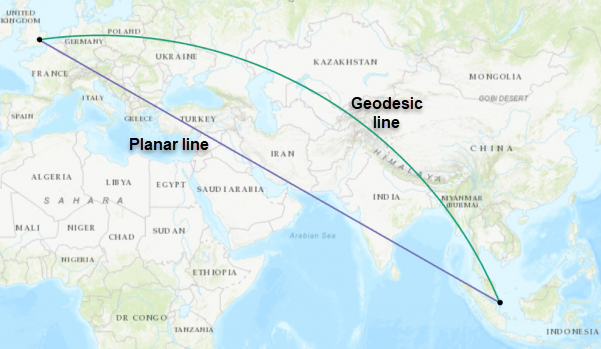English

# FAQ: Why does the Measure tool show a different distance than that set by the Buffer tool?

## Question

Why does the Measure tool show a different distance than that set by the Buffer tool?

The measurement value set by the Buffer tool uses the input data layer coordinate system. The measurement value set by the Measure tool uses the data frame coordinate system. If the input data layer and the data frame use different coordinate systems, a different value is shown although both tools are measuring the same distance.Even if the data frame and the input data layer use the same coordinate system, the readings may vary if the Measure tool uses the planar method while the Buffer tool uses the geodesic method. The geodesic method is used in a geographic coordinate system (GCS) and it factors in the earth's ellipsoid shape in buffer calculations when measuring two points on a globe. The geodesic line represents the shortest distance between two points according to the earth’s curvature.

```Note:
In a geographic coordinate system, if the dataset and the data frame use different geographic systems, a geographic (datum) transformation is required. It is important to use the right geographic transformation. Incorrect geographic transformation causes the coordinates to sway from the original location by a range of a few hundred meters. For more information, refer to ArcMap Web Help: Choosing an appropriate transformation.```

The planar measurement is used in a projected coordinate system (PCS). It uses the Euclidean buffer and 2D Cartesian mathematics to calculate length and area based on the 2D plane of the coordinate system.In some instances, the WGS 1984 Web Mercator projections deliver incorrect buffer measurements regardless of the buffer method and the measurement type used. This is because the WGS 1984 Web Mercator uses a conformal projection that preserves direction and the shape of data but distorts distance on the map. For more information, refer to FAQ: Why are my map, distance and area measurements wrong when using WGS 1984 Web Mercator?

If the Measure tool shows a different distance than that set by the Buffer tool, verify the type of projection, buffer method, and measurement type used. Make sure the following criteria are met: